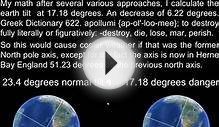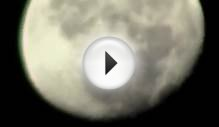# Gravitational pull between Earth and Moon

May 3, 2023F = G m M / r2

in which F is the gravitational force between the two bodies, m and m are their masses, and r is the distance between them, has the same value regardless of which object is thought of as pulling on the other.
In other words, both Laws imply that the force the Earth exerts on the Moon is numerically identical to the force the Moon exerts on the Earth. The only difference is that the Moon pulls the Earth toward the Moon, while the Earth pulls the Moon toward the Earth - that is, the force on each object is toward the other object, and therefore in the opposite direction. (This is usually expressed by saying that the forces are "equal and opposite", but sometimes people think this means they cancel each other, unless it is made clear that the forces are acting on different objects.)

The Basic Effect of the Force Between the Earth and Moon
According to Newton's Second Law of Motion, the Force Law, the effect of a force on an object is to accelerate it in the direction of the force, according to the formula

F = m a

where F is the force applied to the mass m, and is the acceleration, or rate of change of the velocity of the object. As written here the formula does not directly show it, but the force and acceleration are vectors, meaning that they have both magnitude and direction. In this case, since they are the only vectors in the equation, they must have the same direction - that is, the change of velocity must be in the direction of the force, as stated above.
If the Earth and Moon did not exert a force on each other they could each move indepently of the other, but because they do exert a force on each other, their velocities are changed according to the magnitude and direction of each force and their respective masses. Since each is pulled toward the other, the Earth is pulled toward the Moon and therefore a little away from the path it would otherwise follow around the Sun in the absence of the Moon, and the Moon is pulled toward the Earth and therefore a little away from the independent path it would otherwise follow around the Sun in the absence of the Earth. If one or the other did not exist, the remaining object would orbit the Sun in an orbit nearly identical to the path the pair currently follows around the Sun, but since both exist they each follow a path that is roughly the same as their imaginary independent paths, but not quite the same paths as a result of their interaction with each other.
The forces that the Earth and Moon exert on each other must be equal, but that does not mean that the effects of those equal forces are the same, because the two objects have very different masses. The Earth is 81.6 times more massive than the Moon, and as a result a given force will affect (or change) its motion 81.6 times less than it would the Moon. The most obvious effect of the Earth's pull on the Moon is that the Moon orbits the Earth once every 27.3 days, moving in an elliptical path with a size of about 240, 000 miles. The far less obvious effect of the Moon's pull on the Earth is that the Earth also "orbits" the Moon every 27.3 days, with an elliptical path 81.6 times smaller than that of the Moon, or only 3, 000 miles in size.
Of course the Earth can't possibly be orbiting the Moon at a distance of 3, 000 miles while the Moon orbits the Earth at a distance of 240, 000 miles. So the elliptical paths just described cannot be around each other. Instead, what the two bodies actually orbit is a point called the center of mass (or barycenter) of the Earth-Moon system, with an orbital path around that point of 3, 000 miles size for the Earth, and 240, 000 miles size for the Moon. The principle is exactly like that of a see-saw or teeter-totter. If two people of very different weights sit on opposite sides of the balance point (or fulcrum), the heavier person must sit closer to the balance point in inverse proportion to their relative weights. For instance, if the heavier person weighs twice as much as the lighter one, they must sit twice as close to the fulcrum. The balance point is the "center of mass" of the see-saw, just as the barycenter is the balance point of the Earth-Moon system. It is this point that actually moves around the Sun in what we call the orbit of the Earth, while the Earth and Moon each move around the barycenter in their respective "orbits".